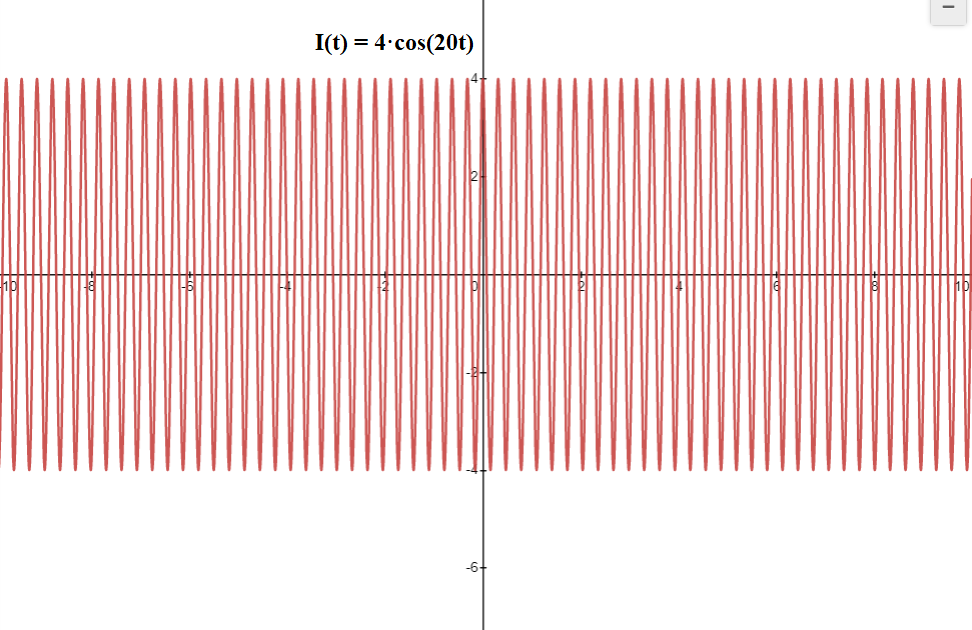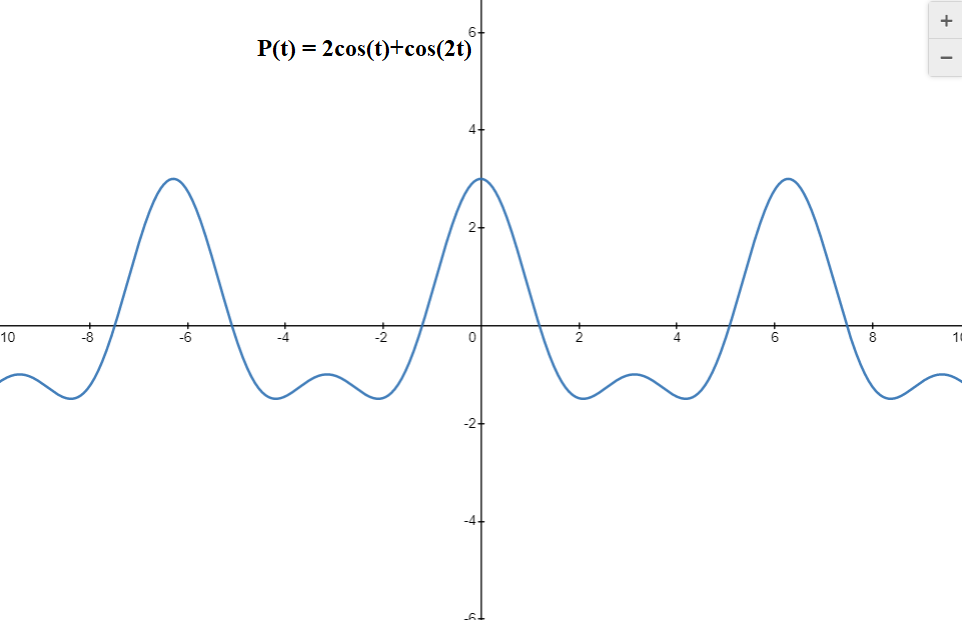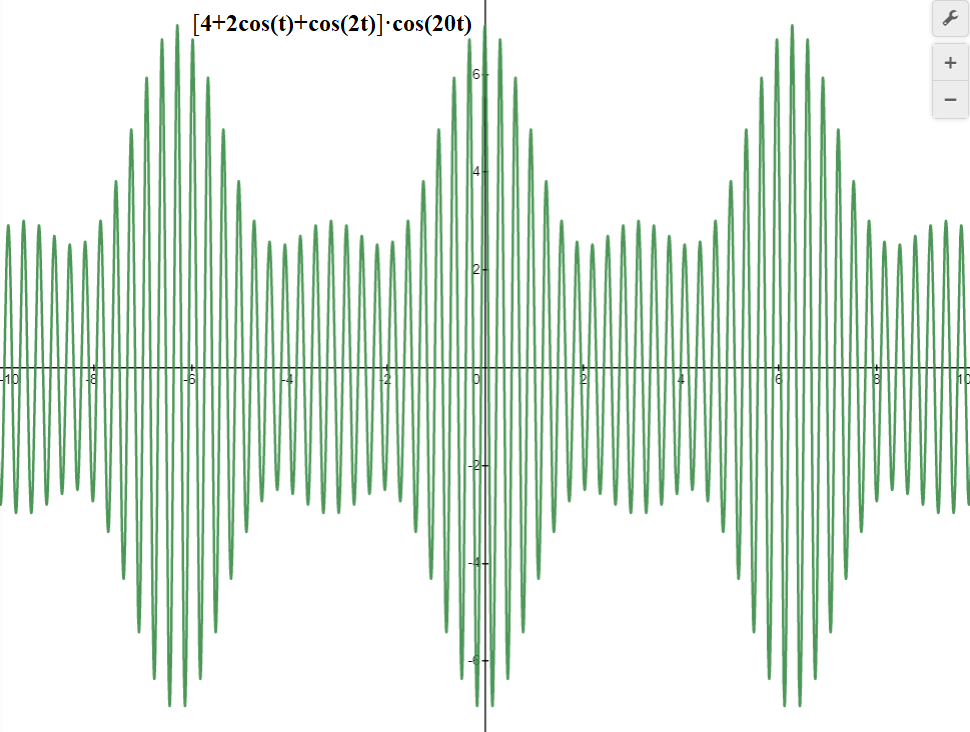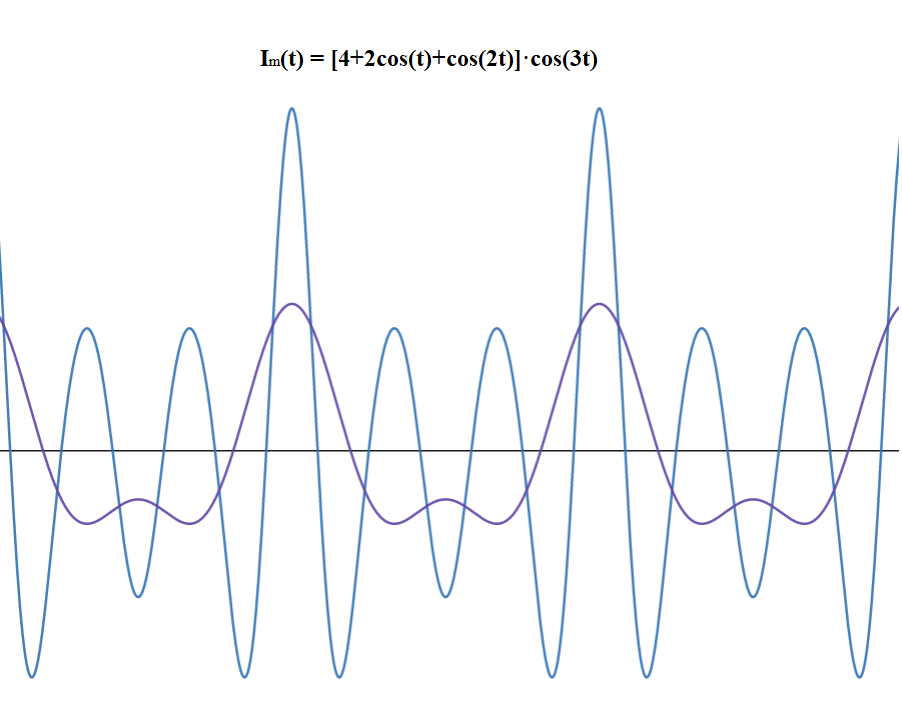Saturday, December 18, 2021

AM Equation: UNIZOR.COM - Physics4Teens - Waves - Radio

Notes to a video lecture on http://www.unizor.com

AM Equation

Let's approach amplitude modulation (AM) from a mathematical standpoint.

High frequency oscillations of the electric current in the LC circuit with an inductor of inductance L and a capacitor of capacitance C can be described by a function
I(t) = Ac·cos(ωc·t)
where
I(t) is the circuit's own oscillation of the current,
Ac is the amplitude of these oscillations,
ωc = 1/√L·C is an angular frequency of these oscillations.

Simple air pressure oscillations resulting from a sound can be expressed by a similar function P(t) = As·cos(ωs·t)
where
P(t) is the oscillation of the air pressure around a source of sound,
As is the amplitude of these air pressure oscillations,
ωs is an angular frequency of these oscillations.

Note that our design requires ωc to be substantially greater than ωs.
Also note that real sound is a combination (superposition) of different overtones with different amplitudes and different angular frequencies and phases, like
P(t) = As1·cos(ωs1·(t+φs1)) +
+ As2·cos(ωs2·(t+φs2)) +
+ As3·cos(ωs3·(t+φs3)) + ...

Amplitude modulation alters the amplitude of the carrier's oscillations by changing it in synch with sound oscillations.
The simplest way to achieve it mathematically is to incorporate the sound waves into an amplitude of carrier's waves:
Im(t) = [Ac+P(t)]·cos(ωc·t)

Here is an example of this type of modulation of a carrier's signal.

Assume, the carrier's high frequency oscillations have an amplitude Ac=4 and angular frequency ωc=20, which can be described by an equation
I(t) = 4·cos(20t)(you can click the right mouse button and open this picture in another tab for better view)

The sound makes air pressure oscillations that combine two different tones, one with an amplitude As1=2 and angular frequency ωs1=1 and another with an amplitude As2=1 and angular frequency ωs2=2, which can be described by the following equation
P(t) = 2·cos(t) + cos(2t)Then the modulated signal can be described by an equation
Im(t) =
=
[4+2·cos(t)+cos(2t)]·cos(20t)
that graphically looks like this:Just as a demonstration of the importance of having a high carrier frequency of a signal to properly represent a sound, here is what the transmitted signal would look like if the LC circuit of a carrier has a frequency comparable to a frequency of sound.

Assume, the carrier's oscillations have an amplitude Ac=4 and angular frequency ωc=3, which can be described by an equation
I(t) = 4·cos(3t)
The sound waves are assumed to be as above
P(t) = 2·cos(t) + cos(2t)
Then the modulated signal can be described by an equation
Im(t) =
=
[4+2·cos(t)+cos(2t)]·cos(3t)
The following graph represents both the sound wave (purple) and modulated signal (blue):As you see, the representation of sound waves by a modulated signal is far from exact. This lower frequency of amplitude modulation cannot be used for transmitting sound.